# Rank of an algebraic group

(diff) ← Older revision | Latest revision (diff) | Newer revision → (diff)

The dimension of a Cartan subgroup of it (this dimension does not depend on the choice of the Cartan subgroup). Along with the rank of an algebraic groupone considers its semi-simple rank and reductive rank, which, by definition, are equal to the rank of the algebraic groupand the rank of the algebraic group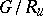respectively, whereis the radical of the algebraic groupandis its unipotent radical (cf. Radical of a group; Unipotent element). The reductive rank of an algebraic groupis equal to the dimension of any of its maximal tori (cf. Maximal torus). The reductive-rank of a linear algebraic groupdefined over a field(and in the case when the groupis reductive (cf. Reductive group) — simply its-rank) is the dimension of a maximal-split torus of it (this dimension does not depend on the choice of a torus; see Split group). If the-rank of a reductive linear algebraic groupoveris zero (is equal to the rank of), then the groupis said to be anisotropic (or split, respectively) over(see also Anisotropic group).

### Examples.

1) The rank of the algebraic group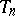of all non-singular upper-triangular square matrices of orderis equal to its reductive rank and equal to; the semi-simple rank ofis zero.

2) The rank of the algebraic group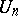of all upper-triangular square matrices of orderwith 1 on the principal diagonal is equal to its dimension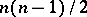, and the reductive and semi-simple ranks ofare zero.

3) The rank of the algebraic group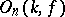of all-automorphisms of a definite quadratic formon an-dimensional vector space over a fieldis equal to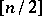, and the-rank of the groupis equal to the Witt index of the form.

If the characteristic of the ground field is 0, then the rank of the algebraic groupcoincides with the rank of its Lie algebra(see Rank of a Lie algebra) and is equal to the minimum multiplicity of the eigen value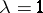of all possible adjoint operators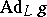(the minimum is taken over all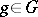). An elementfor which this multiplicity is equal to the rank of the algebraic groupis called regular. The set of regular elements ofis open in the Zariski topology on.

How to Cite This Entry:
Rank of an algebraic group. Encyclopedia of Mathematics. URL: http://encyclopediaofmath.org/index.php?title=Rank_of_an_algebraic_group&oldid=16419
This article was adapted from an original article by V.L. Popov (originator), which appeared in Encyclopedia of Mathematics - ISBN 1402006098. See original article